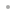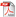MathCode C++MathCode C++FeaturesExamplesQ&ANew in Version 1.4Download ProductManualDownload Product Information SheetBuy OnlineRequest a Free Trial# Q&A

### Technology

Q: How does MathCode work?
MathCode C++ and MathCode F90 take functions written in Mathematica and generate correct C++ or Fortran functions, respectively, and then link them into an executable.

Q: How much speedup can I expect?
MathCode can achieve a speedup of up to 1000 times.

Q: Can MathCode speed up symbolic transformations?
No, in this case you should use the Mathematica function Compile[]. Please contact MathCore support at mathcore@wolfram.com if you have any questions regarding the compilable Mathematica subset of MathCode.

Q: Does MathCode contain a runtime system?
Yes, the runtime system comes together with MathCode and it is linked to your generated C++ or Fortran code. Thanks to this runtime system, you can efficiently perform numerical computations on scalars and matrices and apply many standard Mathematica functions.

Q: Is MathCode compatible with Mathematica application packages?
Yes, but in some cases there might be some conflicts. Please contact MathCore support at mathcore@wolfram.com if you plan to use MathCode together with other Mathematica application packages.

Q: Does MathCode have a type system?
Yes, the user has to add data type information to the variables used in his/her function. This makes it possible to generate very efficient, clear, and straightforward code.

Q: Which data types does MathCode support?
MathCode supports scalars, matrices, and tensors of integer and double and complex numbers.

Q: Are declarations generated?
Yes, as soon as the user supplies data type information for Mathematica variables, it is used in order to generate corresponding variable declarations in C++ or Fortran code. This facilitates very efficient handling of variables.

Q: What is the maximal matrix rank
The maximal matrix rank of MathCode is 4. This means that four-dimensional matrices (K x L x M x N) can be used as a data type.

Q: Is the resulting code readable for humans?
Yes, MathCode C++ and MathCode F90 produce readable C++ and Fortran code, respectively.

Q: How much time does it take to start using it?
The built-in examples make it easy to get started with MathCode, and typically it takes between 3-5 days.

### Licensing

Q: Is there any discount for academic institutions?
Yes, MathCode has a 70% discount for academic institutions.

Q: What is the current version of MathCode?
MathCode C++ 1.4.6 (1.4.7 for Mac OS X Mavericks 10.9) and MathCode F90 1.2.6 are the latest versions of MathCode.

Q: If I currently own an earlier version of MathCode, how do I get an upgrade copy of MathCode?
MathCode has program support and a free update service for 12 months after purchasing a permanent license or an upgrade. After the initial period, this service costs 20% of the price for a new license/year. If you have a valid support agreement and need to receive the latest update, or if you need to renew your support, please contact mathcore@wolfram.com.

### Support

Q: Does MathCore offer MathCode consulting?